Multiple Choice Examination System

1. Database Design and Implementation for General Chemistry

Horea Iustin NAŞCU, Lorentz JÄNTSCHI

Technical University of Cluj-Napoca, Romania

Abstract

The paper presents the main aspects and implementation of the classification system, database design and software, implementation of a multiple choice examination system for general chemistry in order to generate tests for student evaluation. The testing system was used to generate items for multiple choice examinations for first year undergraduate students in Material Engineering and Environmental Engineering from Technical University of Cluj-Napoca, Romania, which all attend the same General Chemistry course.

Keywords

General Chemistry Classification Subjects, Students Evaluation, Multiple Choice Examination, Databases

Introduction

The student evaluation at the end of a semester usually comprises all discussed subjects on course, laboratory, seminars, and projects. When the area of subjects is large, as in general chemistry, a practical idea is to create a multiple choice examination system. The practice is prevalent because multiple choice examinations provide a relative easy way to test students on a large number of topics. More, for large number of students, a classical evaluation system (written evaluation) consumes a considerable amount of time.

Using of a multiple-choice questions evaluation does not exclude the application of other evaluation systems (oral, home papers, en route during laboratory works) and maybe the best idea is to combine all of them in order to give the final mark.

When evaluation does not take place in the same time for all students, the multiple-choice questions tests must be regenerated in order to avoid learning of answers.

The characteristics of a good MCE are well known -. A number of papers on multiple choice randomized examinations in which the order of items (or questions), as well as the order of the possible responses, is randomized independently for each student, have been published -.

By using of a database server features, a testing system which generate tests with 60 multiple choice examination items for general chemistry student level course was build. The tests was applies on first year undergraduate students in Material Engineering and Environmental Engineering from Technical University of Cluj-Napoca, Romania.

Subjects Classification

At beginning of multiple-choice questions test constructing the area of subjects must be classified. A good idea is to start from naturally classification in chapters, sections, and subjects.

According our classification for general chemistry (part one) for material and environment engineering students, nine chapters were chosen: Object of chemistry, Atom structure, Periodic system, Chemical bond, Chemical reactions, States of matter, Thermodynamic, Chemical kinetic, Electrochemistry.

The chapter Object of chemistry contain the sections: Importance of chemistry, Fundamentals, and Stoechiometry calculus. The chapter Atom structure has Quantum mechanic model and The “aufbau” principles. The Periodic system chapter has Historical and Long form sections. The chemical bond chapter has Stable configurations, Ionic bond, Covalent bond, Bond from quantum mechanics point of view, Metallic bond, and Intermolecular bonds sections. The chapter Chemical reactions has Generalities, Acid-base, Precipitation, and Redox sections. The chapter States of matter has Gases, Solids, and Liquids sections. The chapter Thermodynamic has Generalities, U and H, Thermo-chemistry, II-nd principle, III-rd principle, G and F, Equilibrium, Solutions, Chemical potential, Equilibrium in solutions, and Reaction equilibrium sections. The chapter Chemical kinetic has only Generalities section. The chapter Electrochemistry has Electrolysis, Cells, and Ionic equilibriums sections.

More, every section has a number of subjects. The table 1 contains the subjects on sections:

Table 1. General Chemistry Subjects

 Section Subjects Importance of chemistry Object of chemistry, Scientific method Fundamentals Substances, Materials, Elements, atoms and molecules, Symbols, Formulas, Mol, Valence, Isotopes, Atomic mass Stoechiometry Simple, With gases, With materials Quantum mechanic model Bases of quantum mechanics, Orbital, Quantum numbers, Hydrogen atom, Poly-electronic atoms The “aufbau” principles Rules, Examples, Ions configurations History Groups and periods, Forms Long form Important groups, Configurations by groups Stable configurations Examples Ionic bond Examples, Ionic networks Covalent bond Lewis symbols, Lewis examples, Bond rank, Formal charge, Resonance, Complex combinations, Characteristics Bond from quantum mechanics point of view VBM and MOM, Simple bond, Double bond, Non-localized bonding Metallic bond Electronic gas, Bands, Characteristics, Fermi level, Examples Intermolecular bonds Van der Waals bond, Hydrogen bond Generalities about chemical reactions Characteristics, Classifications Acid-base Simple and ionic equations, Hydrolysis Precipitation Solubility, Rules, Equations Redox Oxidation and reduction, Oxidation numbers, Semi-reactions, Reactions – examples Gases Gases laws, Avogadro law, Dalton law, Real gases Solids Generalities, Network types, Atoms per cells, Coordination numbers Liquids Vapor pressure, Vaporizing heat, Other characteristics Generalities about Thermodynamics Fundamentals U and H Principle 0, Principle 1, Sign convention, Enthalpy – relations, Molar heats Thermo-chemistry Laws and generalities, Calculation of reaction enthalpy, Khirchoff equation Second principle Forms of second principle, Types of disorder, Entropy, Calculation of reaction entropy Third principle Calculation of entropy at T G and F Generalities and consequences of second principle, Calculation of reaction free enthalpy Equilibrium Equilibrium constrain, Phases law, Phases diagram for one component Solutions Definitions, rules, classifications, Concentrations, Problems Chemical potential Ideal and real solutions, Definition and equations, Concentration dependence Equilibrium in solutions Solving equilibrium, Gases vs. Liquids – Henry law, Distribution equilibrium, Laws for ideal solutions Reaction equilibrium Homogenous – laws and equations, Homogenous – examples of reaction equilibriums, Heterogeneous – examples of reaction equilibriums, Equilibrium displacement Generalities about chemical kinetic Reaction speed, Catalysts, Activation energy Electrolysis Generalities, Examples of electrolysis Cells Cells examples, Standard potential, Accumulators, Corrosion Ionic equilibriums Dissociation and electrolytes, Acids and bases (Ka and Kb, Lewis), pH, pOH, and pK, Titration and indicators, Buffer solutions, Precipitation reactions, Complex forming reactions

In our evaluation system, every multiple-choice question has assigned a chapter, a section, and a subject.

Database Design

The implementation of multiple-choice questions evaluation system uses a relational database that store chapter, section, and subject names, questions, and answers. A MySQL database server stores the database on vl.academicdirect.ro server.

The structure of the database is depicted in figure 1:

 SubjectsSections id Chapters id fk Semesters id fk data id fk data data data Answers id Questions fk id data fk Correct problem an points qu data

Figure 1. General Chemistry (1) relational database structure

The `Semesters` table store (`data` field) the semesters numbers from study plan which are allocated to General Chemistry course (in our care 1 and 2). The `Semester` table is the parent table for `Chapters` table.

The `Chapters` table store (`data` field) the names of general chemistry studied chapters on every semester (child table of `Semester` table).

The `Sections` table are child table of `Chapters` table and are parent table of `Subjects` table and store General Chemistry sections.

The `Subjects` table are child table of `Sections` table and are parent table of `Questions` table and store General Chemistry subjects.

The `Questions` table are child table of `Subjects` table and are parent table of `Answers` table and store General Chemistry questions.

The `Answers` table is child table of `Questions` table and store at least four answers for every question. More, both `Answers` and `Questions` tables are parents of `Corect` table which store the keys of correct answers for every question.

Software Implementation

Tables creating are of single task type, and are required once, at design time. It not requires dedicated software, can, and was done using phpMyAdmin software.

The database administrative software includes the programs for inserting and modifying questions and answers and is accessible via the address:

The test generation software includes the programs for tests generation and is accessible via the address:

Both pathways are password protected and are manipulated only by the system administrator.

The first page of inserting and modifying interface is in figure 2: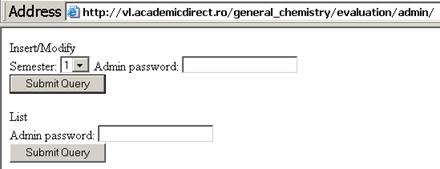Figure 2. Administrative software entering interface

The Insert/Modify option goes to an interface (figure 3) that allow to select a chapter, by using of a select phrase (SELECT * FROM `Chapters`).

By selecting a chapter and pressing the Submit Query button, another program prepares and displays (figure 4) the sections from the selected chapter, by using of a select phrase (SELECT * FROM `Sections` WHERE `fk` = '\$_POST['capitol']').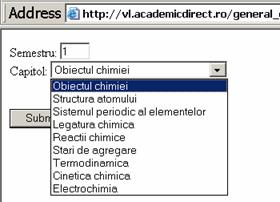Figure 3. Chapter selection in administrative software interface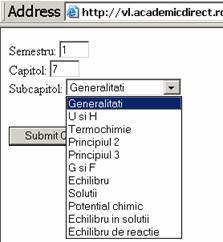Figure 4. Section selection in administrative software interface (example for chapter 7)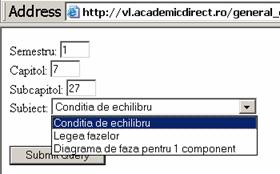Figure 5. Subject selection in administrative software interface (example for section 27)

By selecting a section and pressing the Submit Querry button, another program prepares and displays (figure 5) the subjects from the selected section, by using of a select phrase (SELECT * FROM `Subjects` WHERE `fk` = '\$_POST['subcapitol']').

At the end of selection, an interface let us user to verify the selections (figure 6). Three queries are used:

·        SELECT * FROM `Chapters` WHERE `id` = '\$_POST['capitol']' LIMIT 1;

·        SELECT * FROM `Sections` WHERE `id` = '\$_POST['subcapitol']' LIMIT 1;

·        SELECT * FROM `Subjects` WHERE `id` = '\$_POST['subiect']' LIMIT 1.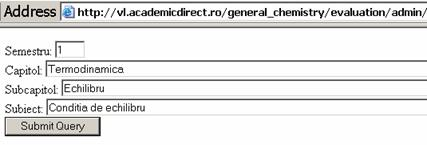Figure 6. Verify selections (example) in administrative software interface

Confirming the selections, the following interface allows both inserting and modifying the questions from database. First part of the interface allows inserting (figure 7).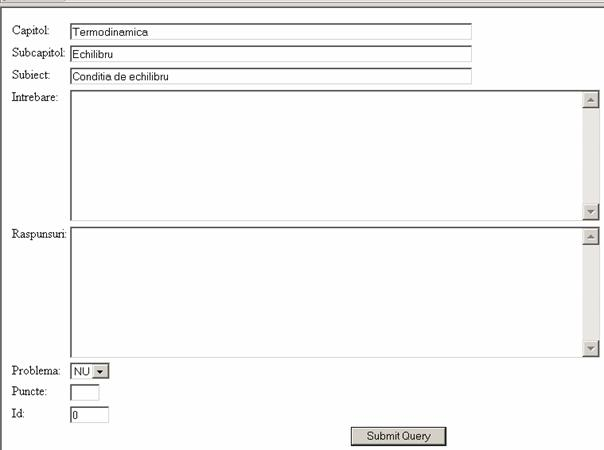Figure 7. Questions inserting in administrative software interface

In order to make an easy to use interface, the answers for a question are placed one by another in a TEXTAREA tag and separator is Enter key (13 ANSI code, \r\n C++ Windows style EANSI character).

Every question can be of problem type (Yes or No), which means that the student must write the resolving algorithm on examination paper and this will be also evaluated separately. More, every question has assigned a difficulty (a number of points from 1 to 5, even if are problem or not).

The modifying interface is in same interface and allows modifying a query and/or answers using key codes from database (figure 8).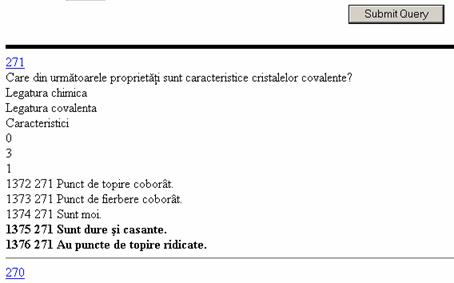Figure 8. Questions modifying pathway in administrative software interface

The first row of question area (271 and 270 in figure 8) is a hyperlink to questions modify interface. The question text follows on the next row(s).

The followings are the chapter, section and subject names, and after codes. The list of answers ends the question area. An answer can be correct (in bold face) or not, and the codes for the answer and related question are in front.

The question key code acts as a hyperlink to questions modify interface (271 in figure 8). By pressing the hyperlink, the question is brought to top of interface, on inserting area (figure 9). Note that the Id input box contains the 271 value (in place of 0, for new insert).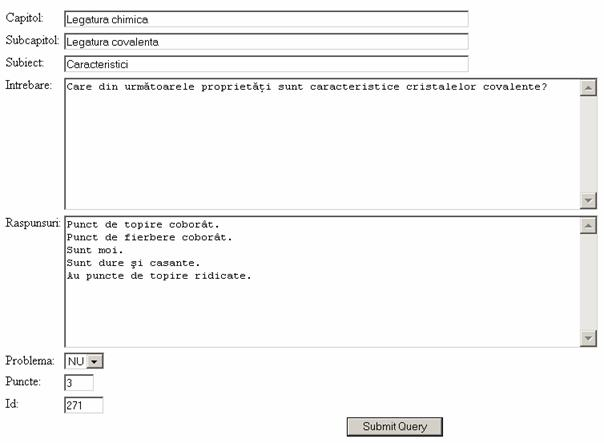Figure 9. A question modifying in administrative software interface

The multiple role of the question insert/modify interface are implemented by using of both POST and GET submitting methods.

The modifying of a question is made through GET method:

if(array_key_exists("id",\$_GET)){

}

in insert/modify program on bringing the question to the TEXTAREA’s and

echo("<A HRef='xx.php?id=".\$qr."'>".\$qr."</A><BR>");

on every question key code from outputted HTML page.

Tests Generation

In order to generate a statistically representative test was count the subjects by chapter which has at least one question into database. In our database, the query produces the following result:

Table 1. Numbers of subjects with questions by chapter

 Chapter 1 2 3 4 5 6 7 8 9 Count(subjects) 11 7 4 14 7 8 17 3 10

If the multiple choice questions test will have 60 questions (as in our case) the distribution of subjects of questions by chapter must follow the distribution of subjects from table 1. Resulted distribution is in table 2.

Table 2. Number of extracted subjects for questions by chapter

 Chapter 1 2 3 4 5 6 7 8 9 Count(subjects) 8 5 3 10 5 6 13 2 8

Other problem can appear from test difficulty. If we accept an about 10% difficulty variation, and the average of questions difficulty (from 1 to 5 points) is 2.7963 (in our case), it result that the average difficulty of test (60 questions) is 167.7 points. Using a ±5% difficulty variation it result that a random generated test can be accepted if the total difficulty (as sum of questions difficulties) are from 160 to 175 points.

The algorithm of tests generation is in figure 10.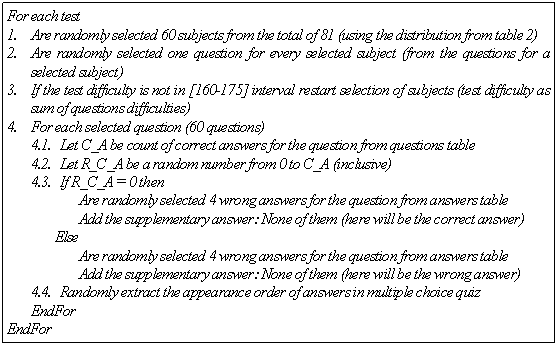Figure 10. The Algorithm for tests generation

Finally, are printed the tests and at the end the evaluation map of correct answers.

A set of queries was used for test generation:

·        List of chapters:

SELECT `data`, `id` FROM `Chapters` ORDER BY `id`

·        List of all subjects which have questions for a chapter:

SELECT `subiect` FROM `Questions` WHERE `capitol` LIKE '\$r1'

·        Primary keys of subjects which have questions for a chapter:

SELECT `id` FROM `Subjects` WHERE `data`='".\$r2."'

·        List of questions for a subject:

SELECT `id`, `puncte`, `problema` FROM `Questions`

WHERE `subiect` LIKE '\$r2' AND `capitol` LIKE '".\$r1."'

·        List of answers for a question:

SELECT `id` FROM `Answers` WHERE `qu`='\$id_q'

·        List of correct answers for a question:

SELECT `an` FROM `Corect` WHERE `qu`='\$id_q'

·        Retrieve the question (for test preparing):

SELECT * FROM `Questions` WHERE `id`='".\$sir[\$i]."' LIMIT 1

·        Retrieve the answer (for test preparing):

SELECT * FROM `Answers` WHERE `id`='".\$sir[\$i]."' LIMIT 1

Results

The multiple choice evaluation tests were applied on two groups of undergraduate students which took the same course one in Materials Engineering (I-ME) and other in Environmental Engineering (I-EE) for the General Chemistry course, first semester of study.

From every group of students a subgroup was extracted on pass examination criteria (I-ME-passed and I-EE-passed). The numbers of points obtained by all tested students by group are in table 3. The underlined values from table 3 represent the pass examination subgroup (which includes also an oral examination). The statistical results for numbers of correct answers obtained after evaluation for all groups and subgroups (table 4) are presented. The obtained scores, on groups and subgroups, allow us to make some statistical interpretations. A good guide for this task is  and the recommendations from the referred book we also use.

Table 3. Experiment results – number of points by groups of students

 Group Points list I-ME 27, 14, 21, 24, 28, 34, 34 I-EE 15, 40, 26, 38, 10, 19, 19, 12, 23, 28, 50, 21, 45, 38, 32, 37, 31, 17, 23, 40, 24, 24, 30, 31, 30, 29, 41, 9

Table 4. Statistical results of evaluation on groups and subgroups

 No Group Students Mean SE 1 I-ME 7 26 2.7 2 I-EE 28 27.93 2.02 3 I-ME-passed 5 29.4 1.99 4 I-EE-passed 15 36 1.68

Let’s note with A the first sample and with B the second sample from the entire population.

The unpooled standard error of the difference of means is the best variance estimator and SE(A,B) is calculated using the formula: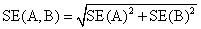,                                                                     (1)

where SE(A) and SE(B) represent the biased standard errors for each group/subgroup.

The degrees of freedom formula of (A, B) population is:(2)

The confidence interval for the difference between means is:

(1-α)CI(A,B) = (M(A)-M(B)) ± t((1-α)/2,df(A,B))∙SE(A,B)                            (3)

where t is the inverse of the Student’s t distribution. By setting to “α” a probability value (such as 5%) we can test if is a difference between the means. If the CI includes the “0” value, we can conclude that with a “α” probability is no difference between means.

To compare the variances, the two-tailed F test (which tests against the alternative that the standard deviations are not equal) is most often used :

F(A,B) = SE(A)2/SE(B)2                                                                                  (4)

If F(α/2, N(A)-1,N(B)-1) < F(A,B) < F(1-α/2, N(A)-1, N(B)-1) then is no difference between variances of samples. By applying the (1)-(4) formulas to the statistical results from table 4, the values from tables 5 and 6 has been obtained.

Table 5. Differences between averages with α=5% probability of wrong

 (A,B) SE(A,B) df(A,B) M(A)-M(B) t(0.025,df(A,B)) 95%CI(A,B) Difference (1,2) 3.37 13.65 -1.93 2.53 [-10.5, 6.6] No (1,3) 3.35 9.90 -3.4 2.69 [-12.4, 5.6] No (1,4) 3.18 10.85 -10 2.63 [-18.3, -1.6] Yes (2,3) 2.83 14.25 -1.47 2.51 [-8.6, 5.6] No (2,4) 2.63 40.19 -8.07 2.33 [-14.2, -2.0] Yes (3,4) 2.61 10.25 -6.6 2.63 [-13, 0.3] No*

*May be Yes

Table 6. Differences between variances with α=5% probability of wrong

 (A,B) F(A,B) F(0.975,N(A)-1,N(B)-1) F(0.025,N(A)-1,N(B)-1) Difference (1,2) 1.79 0.20 2.92 No (1,3) 1.84 0.16 9.20 No (1,4) 2.58 0.19 3.50 No (2,3) 1.03 0.30 8.48 No (2,4) 1.45 0.42 2.76 No (3,4) 1.40 0.12 3.89 No

Discussions

The use of multiple choice examination items tests allow to cover a large number of subjects from the entire course. In our case, the test covers always all chapters and a percent of 74% from the total number of subjects (60 from 81).

The extraction of a reduced number of questions (60 from 270 questions in our database), a reduced number of questions (240 from 1376 answers in our database) randomization of questions order and answers belonging to a question order make almost impossible the learning of answers. Even with all questions and answers published , the students obtained results demonstrate this assumption.

The results open an interesting discussion, sustained by a 5% probability of wrong. Between entire groups of students is no difference between averages and variances of correct responses in the tests (the (1,2) pair of groups from tables 5 and 6). It result that students from both groups assimilates the overall general chemistry knowledge in same manner.

More, the average level of knowledge of subgroup of students from I-ME which passed the exam is no significantly different from the entire group (the (1,3) pair) and also not significantly different from the I-EE entire group (the (2,3) pair). The subgroup of students from I-EE which passed the exam is significantly different in terms of average level of general chemistry knowledge relative to both I-ME and I-EE entire groups (the (1,4) and (2,4) pairs). In terms of variance, the subgroup of students from I-EE which passed the exam is not significantly different from all others. This can lead to the conclusion that the students which passed the exam from entire I-EE group have higher average level of general chemistry knowledge than almost all others (note that the (3,4) average comparison from table 5 is close to be significantly different, which is a remarkable fact, because subgroup 3 is composed from the students which also pass the examination from I-ME group).

Conclusions

The use of MySQL database server combined with an easy to use programming language (such as PHP) and output interface (such as HTML) make the task of multiple choice examination system creation an easy one. More, any update is easy to make.

A statistical interpretation of multiple choice examination tests results can reveal interesting aspects about the level of knowledge on different groups of students, which can lead to educational issues applicable on practical works and seminaries.

References

. The influence of assessment method on students' learning approaches: Multiple choice question examination versus assignment essay, Scouller K., Higher Education, 35, 4, 1998, p. 453-472.

. Boss J., "Multiple-Choice" Examination (Correspondence), The Lancet, 282, 7322, 1963, p. 1382.

. Newble D. I., Elmslie R. G., A New Approach to the Final Examinations in Medicine and Surgery, The Lancet, 318, 8245, 1981, p. 517-518.

. McLeod I., Zhang Y., Yu H., Multiple-Choice Randomization, J. Stat. Edu., 2003, 11(1), online: http://www.amstat.org/publications/jse/v11n1/mcleod.html.

. Yip D. Y., Developing a Better Understanding of the Relationship Between Transpiration and Water Uptake in Plants, Journal of Science Education and Technology, 12, 1, 2003, p. 13-19.

. Skiena S., Sumazin P., Shift Error Detection in Standardized Exams, Journal of Discrete Algorithms, 2, 2, 2004, p. 313-331.

. Ganter Phil, BIO 311 Biostatistics, Online Course, http://www.tnstate.edu/ganter/BIO%20311%20CoursePage.html

. Snedecor, G. W., Cochran, W. G., Statistical Methods, Eighth Edition, Iowa State University Press, 1989.

. Naşcu H., Chemistry I - Autoevaluation Questions (in Romanian), 2005, AcademicDirect Publishing House, ISBN 973-86211-9-4.